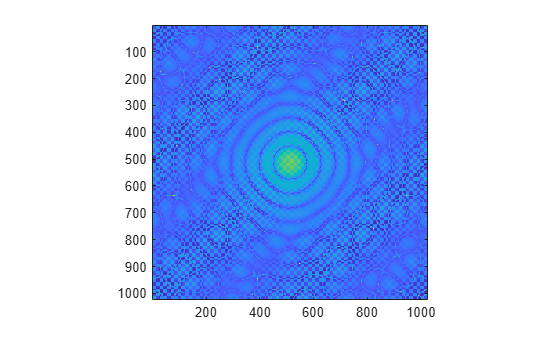2-D Fourier Transforms

The fft2 function transforms 2-D data into frequency space. For example, you can transform a 2-D optical mask to reveal its diffraction pattern.

Two-Dimensional Fourier Transform

The following formula defines the discrete Fourier transform Y of an m-by-n matrix X.

${Y}_{p+1,q+1}=\sum _{j=0}^{m-1}\sum _{k=0}^{n-1}{\omega }_{m}^{jp}{\omega }_{n}^{kq}{X}_{j+1,k+1}$

ωm and ωn are complex roots of unity defined by the following equations.

$\begin{array}{l}{\omega }_{m}={e}^{-2\pi i/m}\\ {\omega }_{n}={e}^{-2\pi i/n}\end{array}$

i is the imaginary unit, p and j are indices that run from 0 to m–1, and q and k are indices that run from 0 to n–1. The indices for X and Y are shifted by 1 in this formula to reflect matrix indices in MATLAB®.

Computing the 2-D Fourier transform of X is equivalent to first computing the 1-D transform of each column of X, and then taking the 1-D transform of each row of the result. In other words, the command fft2(X) is equivalent to Y = fft(fft(X).').'.

2-D Diffraction Pattern

In optics, the Fourier transform can be used to describe the diffraction pattern produced by a plane wave incident on an optical mask with a small aperture . This example uses the fft2 function on an optical mask to compute its diffraction pattern.

Create a logical array that defines an optical mask with a small, circular aperture.

n = 2^10;                 % size of mask
M = zeros(n);
I = 1:n;
x = I-n/2;                % mask x-coordinates
y = n/2-I;                % mask y-coordinates
[X,Y] = meshgrid(x,y);    % create 2-D mask grid
R = 10;                   % aperture radius
A = (X.^2 + Y.^2 <= R^2); % circular aperture of radius R
M(A) = 1;                 % set mask elements inside aperture to 1
axis imageUse fft2 to compute the 2-D Fourier transform of the mask, and use the fftshift function to rearrange the output so that the zero-frequency component is at the center. Plot the resulting diffraction pattern frequencies. Blue indicates small amplitudes and yellow indicates large amplitudes.

DP = fftshift(fft2(M));
imagesc(abs(DP))
axis imageTo enhance the details of regions with small amplitudes, plot the 2-D logarithm of the diffraction pattern. Very small amplitudes are affected by numerical round-off error, and the rectangular grid causes radial asymmetry.

imagesc(abs(log2(DP)))
axis imageFowles, G. R. Introduction to Modern Optics. New York: Dover, 1989.